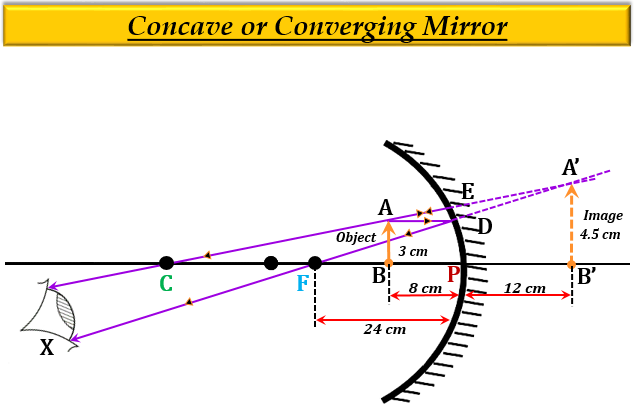# An object 3 cm high is placed at a distance of 8 cm from a concave mirror which produces a virtual image 4.5 cm high:(i) What is the focal length of the mirror?(ii) What is the position of image?(iii) Draw a ray-diagram to show the formation of image.

(i) Given:

Distance of the object from the mirror $u$ = $-$8 cm

Height of the object, $h_{1}$ = 3 cm

Height of the image $h_{2}$ = 4.5 cm

To find: The focal length of the mirror $f$, and the distance of the image $v$ from the mirror $v$.

Solution:

From the magnification formula, we know that-

$m=\frac{{h}_{2}}{{h}_{1}}=-\frac{v}{u}$

Substituting the given values in the magnification formula we get-

$\frac{4.5}{3}=-\frac{v}{(-8)}$

$\frac{4.5}{3}=\frac{v}{8}$

$3v={4.5}\times {8}$

$v=\frac{{4.5}\times {8}}{3}$

$v={1.5}\times {8}$

$v=+12cm$

Thus, the distance of the image $v$ is 12 cm, and the positive sign implies that the image forms behind the mirror (on the right).

Now, from the mirror formula, we know that-

$\frac{1}{f}=\frac{1}{v}+\frac{1}{u}$

Substituting the given values in the mirror formula we get-

$\frac{1}{f}=\frac{1}{12}+\frac{1}{(-8)}$

$\frac{1}{f}=\frac{1}{12}-\frac{1}{8}$

$\frac{1}{f}=\frac{2-3}{24}$

$\frac{1}{f}=\frac{-1}{24}$

$f=-24cm$

Thus, the focal length of the concave mirror $f$ is 24 cm, and the negative sign implies that the focus is in front of the concave mirror (on the left).

(ii) $v=12$ (calculated above).

Hence, the position of the image is 12 cm behind the mirror.

(iii) Ray diagram to show the formation of the image.Updated on: 10-Oct-2022

381 Views